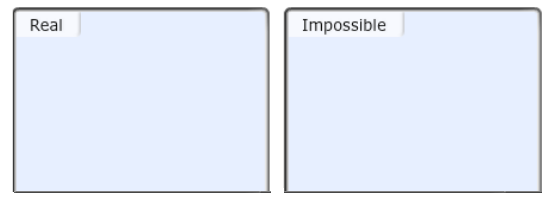# Problem: Which of the following combinations of n and l represent real orbitals and which are impossible? Drag the appropriate items to their respective bins.2s, 5d, 3f, 3s

🤓 Based on our data, we think this question is relevant for Professor Szulczewski's class at UA.

###### Problem Details

Which of the following combinations of n and l represent real orbitals and which are impossible? Drag the appropriate items to their respective bins.

2s, 5d, 3f, 3sWhat scientific concept do you need to know in order to solve this problem?

Our tutors have indicated that to solve this problem you will need to apply the Introduction to Quantum Mechanics concept. You can view video lessons to learn Introduction to Quantum Mechanics. Or if you need more Introduction to Quantum Mechanics practice, you can also practice Introduction to Quantum Mechanics practice problems.

What is the difficulty of this problem?

Our tutors rated the difficulty ofWhich of the following combinations of n and l represent rea...as low difficulty.

How long does this problem take to solve?

Our expert Chemistry tutor, Dasha took 1 minute and 35 seconds to solve this problem. You can follow their steps in the video explanation above.

What professor is this problem relevant for?

Based on our data, we think this problem is relevant for Professor Szulczewski's class at UA.